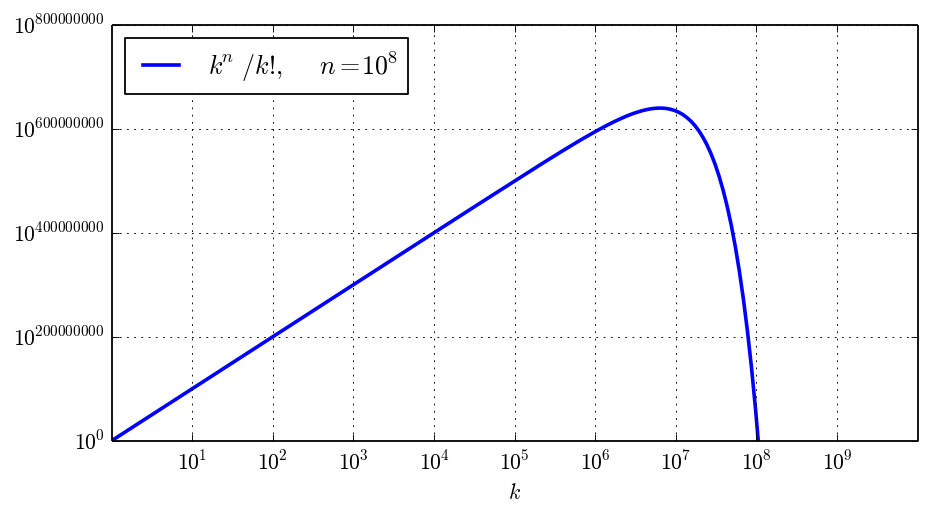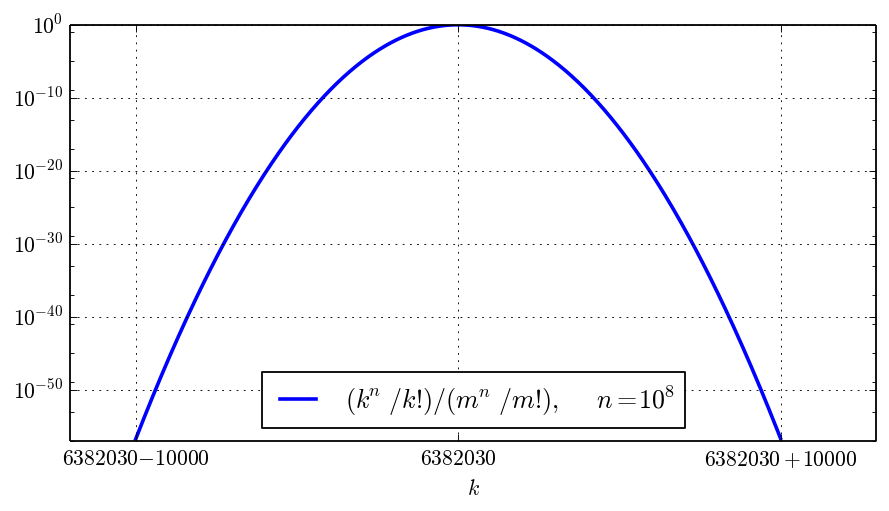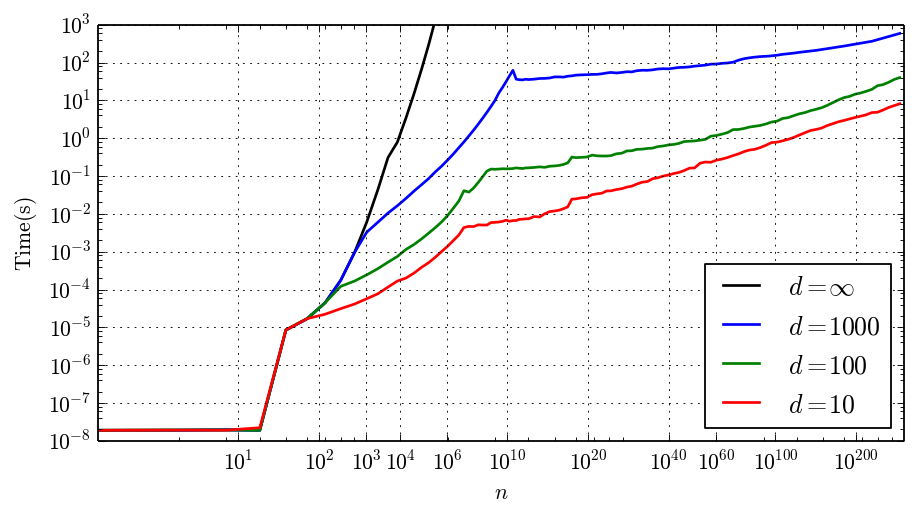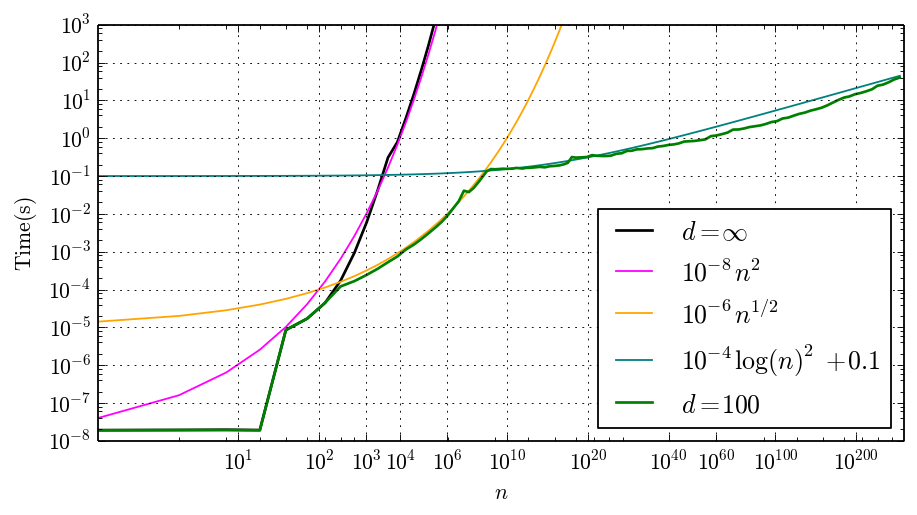# Computing Bell numbers

August 6, 2015

The Bell numbers $B_n$ count the number of ways a set with $n$ elements can be partitioned into disjoint subsets. The integer sequence $B_0, B_1, \ldots$ (OEIS A000110) begins $$1, 1, 2, 5, 15, 52, 203, 877, 4140, 21147, \ldots$$

It is easy to see that $B_n \le n!$ by induction. A partition of the set $A = \{a_1, \ldots, a_{n-1}\}$ can be extended to a partition of the set $A \cup \{a_n\}$ in at most $n$ different ways: we can either add $a_n$ to one of the existing subsets (of which there are at most $n-1$), or create a new subset only containing $a_n$. Indeed, the Bell numbers grow almost as fast as this upper bound, which has size $\log n! = n \log n - n + O(\log n)$ by Stirling's formula. The Wikipedia article lists various asymptotic estimates, such as $$\frac{\log B_n}{n} = \log n - \log \log n - 1 + \frac{\log \log n}{\log n} + \frac{1}{\log n} + \frac{1}{2}\left(\frac{\log \log n}{\log n}\right)^2 + O\left(\frac{\log \log n}{(\log n)^2} \right).$$ and the even more precise $$B_n \sim n^{-1/2} (n/W(n))^{n+1/2} \exp\left(n/W(n) - n - 1\right)$$ where $W(x)$ is the Lambert W-function which solves $W(x) e^{W(x)} = x$.

How can we calculate $B_n$ efficiently when $n$ is very large? I have written extensively about computing the function $p(n)$, which counts the number of integer partitions of $n$ (see my paper in LMS J. Comp. Math, chapter 5 in my thesis, and my notes about computing the exact value of $p(10^{20})$). In the case of integer partitions, it is possible to compute $p(n)$ in time $n^{1/2+o(1)}$, which is quasi-linear in the size of the result. Counting set partitions currently seems to be a harder problem: the best algorithms available compute $B_n$ in time $n^{2+o(1)}$, which is quasi-quadratic in the size of the result.

## Calculating Bell numbers exactly

The easiest way to compute Bell numbers is to use a number triangle resembling Pascal's triangle for the binomial coefficients. The Bell numbers appear on the edges of the triangle. Starting with 1, each new row in the triangle is constructed by taking the last entry in the previous row as the first entry, and then setting each new entry to its left neighbor plus its upper left neighbor:

1
1    2
2    3    5
5    7   10   15
15  20   27   37   52
...


This algorithm has $n^{3+o(1)}$ complexity since we perform $O(n^2)$ additions of numbers with $n^{1+o(1)}$ bits each. This is not optimal asymptotically, but since it only involves additions, it actually beats cleverer methods until $n$ gets quite large. This method is more than enough for any real-world use. You can safely stop reading here!

To compute $B_n$ in just $n^{2+o(1)}$ time, we can use Dobinski's formula $$B_n=\frac{1}{e}\sum_{k=0}^\infty \frac{k^n}{k!}$$ or what might be called the finite Dobinski formula $$B_n = \sum_{k=1}^n \frac{k^n}{k!} \sum_{j=0}^{n-k} \frac{(-1)^j}{j!}.$$ Either formula requires similar computational effort: the infinite series requires just slightly more than $n$ terms to determine $B_n$ exactly. The bulk of the work with either formula is to compute all the powers $k^n$. The factorials can be amortized using binary splitting (recursively dividing the sum in half and factoring out the partial products for the factorials), but since the powers remain, this results in a modest overall improvement.

We can hope to gain a little more by sieving: we compute $p^n$ using binary exponentiation when $p$ is prime, and compute $k^n = q^n r^n$ when $k = qr$ is composite. This has the drawback that the powers have to be stored in memory, taking up $n^{2+o(1)}$ bits of space, which soon gets very large.

We can actually use the finite Dobinski formula to compute a Bell number modulo a prime $p$ with $n < p < n^{1+o(1)}$ in only $n^{1+o(1)}$ time. In fact, this allows us to compute $B_n$ efficiently using a multimodular algorithm: we compute each $B_n$ modulo $p_i$ for a set of primes with $B_n < p_1 p_2 \cdot p_N$, and then reconstruct $B_n$ using the Chinese remainder theorem. This still has $n^{2+o(1)}$ complexity, but there are some advantages compared to using Dobinski's formula directly with large integers:

• Arithmetic modulo word-size primes can be done efficiently.
• The memory required for sieving becomes $n^{1+o(1)}$ instead of $n^{2+o(1)}$.
• The algorithm is easily parallelized by processing different primes on different cores.

Dobinski's formula is not the end of the story. Like the number triangle algorithm, it takes $n^{3+o(1)}$ to compute the first $n$ Bell numbers simultaneously. We can use either the ordinary generating function (OGF) $$\sum_{k=0}^{\infty} \frac{x^k}{\prod_{j=1}^k (1-jx)} = \sum_{k=0}^{\infty} B_k x^k$$ or the exponential generating function (EGF) $$e^{e^x-1} = \sum_{k=0}^\infty \frac{B_k}{k!} x^k$$ to compute the first $n$ Bell numbers simultaneously in $n^{2+o(1)}$ time. The idea is to evaluate the left-hand formula using asymptotically fast power series arithmetic (using FFT for multiplication) and then read off the coefficients on the right-hand side. For the best complexity, the sum in the OGF should be evaluated binary splitting, and the exponential in the EGF should be evaluated using Newton iteration. The complexity with either the EGF or the OGF is quasi-optimal, since each Bell number is computed in $n^{1+o(1)}$ time. However, there doesn't seem to be a way to compute just one of the numbers any faster than computing all of them at once.

The EGF or OGF can also be used to compute the first $n$ Bell numbers simultaneously modulo a small prime in only $n^{1+o(1)}$ time. Combining the EGF with multimodular evaluation to compute the full integers $B_0, \ldots, B_n$ simultaneously is quite attractive since it avoids factorial-sized denominators we get when doing arithmetic in $Q[[x]]$.

I implemented most of the algorithms described above in FLINT back in 2011.

There are also default functions in FLINT that automatically choose the (roughly, depending on hardware) fastest algorithm.

The following table shows how many seconds FLINT takes with the respective algorithm on my laptop:

 n vec_recursive vec_multi_mod bsplit multi_mod 10 0.000000013* 0.0000076 0.0000039 0.0000059 100 0.00011 0.00033 0.000031 0.000094 1000 0.070 0.30 0.0060 0.0093 10000 87 118 3.1 1.2 100000 - - 858 164

Note that the timings for the first two algorithms (vec_recursive and vec_multi_mod) are for computing the first $n$ Bell numbers simultaneously, while the last two are for computing $B_n$ alone. The asterisk (*) indicates that the code actually cheats here by using a lookup table when $n < 26$.

We see that the number triangle algorithm beats the EGF (vec_multi_mod) for reasonable values of $n$. The number triangle algorithm has worse asymptotic complexity but much lower overhead.

I did not implement parallelization of the multi_mod algorithms. This should give close to a 2x speedup on my dual core processor.

There is no implementation based on the OGF in FLINT, but I've tested this algorithm, and it has almost identical speed to the EGF with multimodular evaluation.

## Calculating Bell numbers approximately

Now assume that, instead of computing the exact value of $B_n$, we are happy with the leading $d$ digits, where $d$ for example might be 10, 100 or 1000. Clearly, if we consider d fixed, the cost of approximating $B_n$ by summing the first $n$ or so terms in Dobinski's formula is $n^{1+o(1)}$. All terms are positive, so we can safely compute the sum in floating-point arithmetic with a few guard bits to account for rounding errors.

But we can do much better than this!

An application of Stirling's formula shows that the terms $k^n / k!$ peak around $k = m$ where $n \approx m \log(m)$ (in other words, $m \approx n / W(n)$). To compute a $d$-digit numerical approximation of $B_n$, we only need to add the terms of magnitude within $10^{-d}$ of $m^n / m!$. A detailed analysis shows that about $O(n^{1/2})$ terms are needed if we consider $d$ fixed (see De Bruijn, Asymptotic methods in analysis).

The following picture shows the global magnitude of the terms, with the example value $n = 10^8$:The following picture zooms in near $m = \lfloor n / W(n) + 1/2 \rfloor = 6382030$, showing that the effective width around the peak is of order $n^{1/2} = 10000$ (the horizontal scale is linear):Thus, for a precision of $d$ digits where $d$ is much smaller than $\log B_n$, computing $\sum_{k=a}^{b-1} k^n / k!$ instead of $\sum_{k=0}^{b-1} k^n / k!$ with a well-chosen $a$ reduces the amount of work from $O(n)$ to $O(n^{1/2})$ (we also need the numerical value of $a!$, but this is easy to compute using Stirling's series for the gamma function). This is a significant improvement if $n$ is large. Can we do even better?

The analytic function $T(z) = z^n / z! = z^n / \Gamma(z+1)$ can be expanded in a Taylor series around the point $z = m$. We can approximate $\sum_{k=m-r}^{m+r} k^n / k!$ by a truncation of the Taylor series and change the order of summation to obtain $$\sum_{x=-r}^r T(m+x) \approx \sum_{x=-r}^r \sum_{k=0}^{N-1} \frac{T^{(k)}(m)}{k!} x^k = \sum_{k=0}^{N-1} \frac{T^{(k)}(m)}{k!} \sum_{x=-r}^r x^k.$$ The nice thing about the formula on the right is that the effort to evaluate it depends on $N$ and not on $r$, thanks to Faulhaber's formula $$\sum_{x=a}^b x^k = \frac{B_{k+1}(b+1) - B_{k+1}(a)}{k+1}$$ where $B_{k+1}(t)$ denotes a Bernoulli polynomial (not a Bell number).

It is not too hard to give an explicit error bound for the Taylor series approximation, allowing us to select $N$ rigorously for a desired precision $d$. If we have a bound for $T(z)$ on a disk ${|z-m| \le R}$ where $r < R$, then we can use one of the best theorems in mathematics, Cauchy's integral formula, to bound the derivatives $T^{(N)}(m), T^{(N+1)}(m), \ldots$, and in turn the Taylor series remainder.

Let $$T(z) = \exp(F(z)), \quad F(z) = n \log(z) - \log \Gamma(z+1).$$ Clearly, $|T(z)| \le \exp(|F(z)|)$. We have $$\sup_{|z-m|\le R} |F(z)| \le |F(m)| + R \sup_{|z-m|\le R} |F'(z)|.$$ However, directly bounding $$F'(z) = \frac{n}{z} - \psi(z+1)$$ does not work, since there is catastrophic cancellation ($F'(z) \approx 0$ holds by construction when $z \approx m$). Instead we proceed to the second derivative $$F''(z) = -\frac{n}{z^2} - \psi^{(1)}(z+1).$$ Integrating twice gives $$\sup_{|z-m|\le R} |F(z)| \le |F(m)| + R |F'(m)| + \tfrac{1}{2} R^2 \sup_{|z-m|\le R} |F''(z)|.$$ Since $|\psi^{(1)}(z+1)| \le 1 / \operatorname{re}(z)$ holds for $\operatorname{re}(z) \ge 0$, we obtain the following bound: for $0 \le R < m$, $$\sup_{|z-m|\le R} |T(z)| \le C |T(m)|$$ where $$C = \exp\left(R |F'(m)| + \frac{1}{2} R^2 \left(\frac{n}{(m-R)^2} + \frac{1}{m-R}\right) \right).$$ Plugging this into the Cauchy integral formula, and using the geometric series bound, we obtain $$\sum_{x=-r}^r T(m+x) = \left[\sum_{k=0}^{N-1} \frac{T^{(k)}(m)}{k!} \sum_{x=-r}^r x^k\right] + \varepsilon \quad |\varepsilon| \le \frac{(2r+1) C T(m) B^N}{1 - B}, \quad B = r / R.$$

It remains to choose $R$ so that convergence is reasonably quick. For example, $R = 10r$ yields one digit for each added term. However, this has to balanced against the fact that $C$ becomes very large when $R$ is large. For best performance, we should choose $R$ so that, for example, $C \le 10^{d/2}$ where $d$ is the number of wanted digits.

Unfortunately, it turns out that $r$ is so large that $C$ cannot be made acceptably small. The algorithm just doesn't work. However, if we divide the summation interval $[m-r, m+r]$ into just a few shorter intervals, say of width $r / 10$ or $r / 30$, and perform the Taylor expansion on each subinterval, it turns out that the $C$ for each subinterval becomes acceptably small, and the algorithm suddenly works beautifully!

The trick of interchanging the order of summation and using Bernoulli polynomials to evaluate power sums gives us an algorithm for approximating Bell numbers whose running time essentially depends on the precision $d$ and not on $n$. In reality, the running time is not quite independent of $n$. We have to work with numbers with at least $O(\log n)$ bits of precision, so the complexity as a function of $n$ should really be something like $\log^{O(1)} n$. This is a massive improvement over the $n^{1/2}$ direct summation, not to mention over the $n^{2+o(1)}$ exact computation of $B_n$ (assuming that all we need is a numerical approximation)!

## Implementation in Arb

I implemented Bell numbers in Arb in this commit. Given an fmpz_t bignum $n$ and a precision $p$ in bits, the function arb_bell_fmpz computes an interval enclosure for $B_n$ of approximate width $2^{-p} B_n$.

The code first determines whether it is faster to call FLINT for the exact value. Otherwise, it evaluates $B_n = (\sum_{k=a}^{b-1} T(k) + \varepsilon) / e$ with a rigorous error bound for $\varepsilon = \sum_{k=0}^{a-1} T(k) + \sum_{k=b}^{\infty} T(k)$.

The code first uses ternary search to locate an index $k = m$ where the term $T(k) = k^n / k!$ approximately is maximal, and then uses binary search to find cutoffs $a < m < b$ such that $\sum_{k=0}^{a-1} T(k)$ and $\sum_{k=b}^{\infty} T(k)$ both are of order $2^{-p} T(m)$. Selecting $a, m, b$ can be done more efficiently than the way it is implemented right now, but I just wanted something that works to begin with.

I implemented two functions for computing the sum $\sum_{k=a}^{b-1} k^n / k!$, where $a, b$ may be bignums. The first implementation uses direct summation (with binary splitting to make the factorials slightly cheaper at high precision), performing $O(b-a)$ floating-point operations; that is, $O(n^{1/2})$ floating-point operations when $n$ is large. The second implementation uses the Taylor series method described above with $\log^{O(1)} n$ complexity.

It was slightly tricky to implement the Taylor series algorithm, because several parameters have to be chosen, including the following:

• The number of times to subdivisions of the initial interval to get a reasonably small value for $r$ and thus for $C$.
• The ratio $r / R$.
• The number of terms $N$ of the Taylor series.
• The working precision used to evaluate $\sum_{k=0}^{N-1} \frac{T^{(k)}(m)}{k!} \sum_{x=-r}^r x^k$ to ensure that the result is accurate to $d$ digits despite rounding errors.

I don't see an easy way to select all these parameters optimally a priori, since they depend on each other in a complicated way. The working precision is particularly difficult to choose since it depends on details in the underlying algorithms for the gamma function, for multiplication of power series, and so on. My solution was to always pick the ratio $r / R = 1/8$ (giving three bits per term) and selecting both the number of subdivisions and the working precision adaptively:

• Given an interval $[a,b-1]$ with midpoint $m$, we check if the constant $C$ for this interval is reasonably small. Otherwise, we call the function recursively to evaluate the sum on $[a,m-1]$ and $[m,b]$, and add the results.
• If $C$ is acceptable, we pick $N$ accordingly and then evaluate the fixed formula $\sum_{k=0}^{N-1} \frac{T^{(k)}(m)}{k!} \sum_{x=-r}^r x^k$. Here we double the precision until the result is accurate to $d$ digits.

It remains to decide when to use which implementation to compute $\sum_{k=a}^{b-1} T(k)$. Some experimentation shows that the Taylor series is faster than direct summation roughly when $$n > 2^{12} p^2$$ where $p$ is the precision in bits. For example:

• To compute a 10-digit approximation of $B_n$, the Taylor series is faster roughly when $n$ is greater than 5 million.
• To compute a 100-digit approximation of $B_n$, the Taylor series is faster roughly when $n$ is greater than 500 million.
• To compute a 1000-digit approximation of $B_n$, the Taylor series is faster roughly when $n$ is greater than 50 billion.

The following plot shows the time arb_bell_fmpz takes to compute $B_n$ to $d$ digits where $d$ is 10, 100, 1000 and $\infty$ (computing $B_n$ exactly).Note that the vertical axis has a log scale and the horizontal axis has a log log scale. You can clearly see where the algorithm changes. Up to $n = 26$, we get instant results since a lookup table is used. Then the exact algorithm with $n^{2+o(1)}$ complexity kicks in, and the time increases rapidly with $n$. Around $d \approx n \log n$ where $B_n$ becomes larger than $d$ digits, the $d = 10, 100, 1000$ curves break off from the $d = \infty$ curve by switching to the $n^{1/2+o(1)}$ algorithm. Finally, around the billion-$n$ mark, switching to the $\log^{O(1)} n$ algorithm drastically slows down the growth in running time. In the following plot, I've put in mathematical curves that roughly match the empirical timing data for $d = \infty$ and $d = 100$:To demonstrate that the code works, here are some large Bell numbers with 50-digit precision. The first value takes 0.0018 seconds to compute; the last 0.14 seconds.

$B_{10^5} =$ 1.0433942425429389984540246838845160786245861774676 · 10364471 ± 3.29 · 10364421

$B_{10^6} =$ 6.9407979938401739982227098407865685636554898570286 · 104547585 ± 2.36 · 104547535

$B_{10^7} =$ 4.3145155655649390291431304090943630466481496281332 · 1054670462 ± 5.72 · 1054670411

$B_{10^8} =$ 1.0661323224103766871234871127158157404496071219044 · 10639838112 ± 2.08 · 10639838062

$B_{10^9} =$ 2.6930773812723249433116475845718644555421493748165 · 107338610158 ± 2.50 · 107338610108

$B_{10^{10}} =$ 5.1453972928520420466420608273749029965573268638547 · 1082857366966 ± 9.28 · 1082857366915

$B_{10^{20}} =$ 5.3827011317628161073953431454940317253902192049701 · 101794956117137290721328 ± 1.58 · 101794956117137290721278

Finally, here is $B_{10^{100}}$, the number of partitions of a set with 1 googol elements, to 1000-digit precision:

2.937547415006984869398920080106574949387725148180178490086522814709141082608913334784314134261851806
2232888949657946267157517765591827437575568527323435768792618847167888789438948060302478614199779726
3678257759505994042279245062445753647863874788822306413960438998857329199453620537788749942016823439
6826778585822533942208369004319687423225479298171524242007012081418958404496161123201363892701142452
0592967211088076726689116500153640131057718252616451791806734296741516356176155638789662447036908840
7386274539020941419887853895977737193023441786354602380530218286706381521744667874212992728960187602
0900512672752037690916240689650852398468230120896448383085739867131963399875467499177815304267568743
4662852459854922316072421122078373333676860456717485594671823500441075731431468000467316898340014880
0563499537605408024020518232971387570187255225724367819000339133679365822937731151484061913797597293
9036570242425082172677880169805458277444328503091835330854941844204279433189349120410802200732718571
· 10972157574857696235378663027434211359218006858504930450816134076178889687987618389929416815288755835629
± 4.37 · 10972157574857696235378663027434211359218006858504930450816134076178889687987618389929416815288755834629

The 1000-digit approximation of this number takes 150 seconds to compute, while computing 10 digits of the same number takes less than 1 second (see the plot above). It is fun to note that the asymptotic formula $B_n \sim n^{-1/2} (n/W(n))^{n+1/2} \exp\left(n/W(n) - n - 1\right)$ suggests the value

2.944... · 1010972157574857696235378663027434211359218006858504930450816134076178889687987618389929416815288755835629

which has the correct exponent but just two correct leading digits! The asymptotic formula actually gives over 100 correct digits of $\log B_{10^{100}}$, but most are eaten by the 102-digit exponent.

## Conclusion

To recap: I added code to Arb for computing numerical approximations of Bell numbers, with arbitrary precision and rigorous error bounds. The implementation automatically selects between different algorithms:

• Exact evaluation (via FLINT) with complexity $n^{2+o(1)}$ when the precision is about as large as $\log B_n$.
• Direct summation of the dominant terms in the infinite series (requiring $n^{1/2+o(1)}$ work at a fixed precision).
• Approximation of the sum using Taylor series approximation of the terms and fast evaluation of power sums via Bernoulli polynomials (requiring $\log^{O(1)} n$ work at a fixed precision).

I doubt this code has any real-world use, but it was a fun exercise to do. I don't have much interest in Bell numbers as such, but rather in the algorithms. As usual, looking at a concrete problem helps one understand the tools one has available to attack harder problems. In this case, several Arb features are being used:

• The support for easily working with variable-precision approximations of real numbers and automatically tracking their error bounds.
• The support for automatic differentiation of transcendental functions (such as the gamma function), allowing us to work with the terms of a series as analytic functions.
• The support for arbitrary-precision exponents, allowing us to approximate mammoth numbers like $B_{10^{100}}$. We could modify the algorithm to compute $\log B_n$, making large exponents unnecessary, but this would be more work.

While trying to generate benchmark data for this blog post, I discovered (and fixed) a small bug in the exponential function (arb_exp) which always caused an interval with low precision to be returned when computing exponentials of very large numbers (exceeding $10^{100}$). This bug first manifested itself as a failure to converge to full accuracy when trying to compute the test value $B_{2^{470}}$ to 1000 digits.

In 2009, I wrote about computing Bell numbers in mpmath. The code in Arb runs orders of magnitude faster even for moderately large values like $n = 10^5$, and an input like $n = 10^{20}$ is completely infeasible with the mpmath implementation. It might be interesting to extend the algorithm in Arb to cover the more general "polyexponential function" $$E_s(z) = \sum_{k=1}^{\infty} \frac{k^s}{k!} z^k$$ which is implemented in mpmath.

A challenge to the reader: can you come up with a faster way to compute Bell numbers (approximately or exactly)? How accurately can you approximate $B_n$ using asymptotic expansions, if you use high-order correction terms? Can you do this with effective, rigorous error bounds?